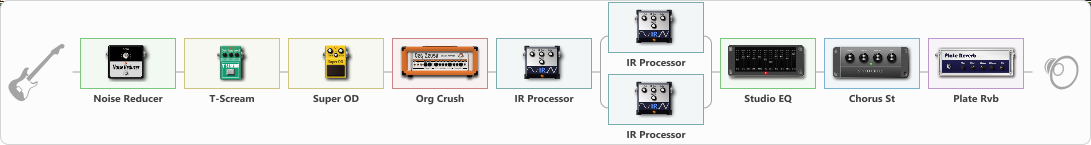# Metallica The Black Album tone

Discussion in 'ToneLib-GFX presets' started by Tony the Metalhead, Jan 7, 2022.

1. ### Tony the MetalheadActive Member

Metallica The Black Album tone

Preset name: RattleSnake

Updated

Effects chain:Effect: "Noise Reducer" (Dynamics / Filter), active - "yes"
{
"Sens" = 70
"Mode" = Hard
}

Effect: "T-Scream" (Overdrive / Distortion), active - "yes"
{
"Drive" = 10
"Tone" = 40
"Level" = 10
}

Effect: "Super OD" (Overdrive / Distortion), active - "yes"
{
"Drive" = 40
"Tone" = 40
"Level" = 30
}

Effect: "Org Crush" (Amp simulators), active - "yes"
{
"Gain" = 85
"Bass" = 30
"Middle" = 5
"Treble" = 60
"Presence" = 55
"Master" = 70
"Level (dB)" = 2
}

Effect: "IR Processor" (Cabinets), active - "yes"
{
"IR" = sandman_stereo_IR
"Low Cut (Hz)" = 250
"Hi Cut (kHz)" = 20.0
"Mix" = 100
"Level (dB)" = 0
}

Effect: "Splitter" (Dynamics / Filter)
{
"A-Bypass" = Off
"A-Pan" = -75
"A-Level" = 55
"B-Bypass" = Off
"B-Pan" = 75
"B-Level" = 55

'A' branch:
{

Effect: "IR Processor" (Cabinets), active - "yes"
{
"IR" = OD-R112-V30-SM58-P05-20
"Low Cut (Hz)" = 125
"Hi Cut (kHz)" = 20.0
"Mix" = 100
"Level (dB)" = 0
}
}
'B' branch:
{

Effect: "IR Processor" (Cabinets), active - "yes"
{
"IR" = OD-R112-V30-SM58-P09-40
"Low Cut (Hz)" = 125
"Hi Cut (kHz)" = 20.0
"Mix" = 100
"Level (dB)" = 0
}
}
}

Effect: "Studio EQ" (Dynamics / Filter), active - "yes"
{
"31 Hz" = -4
"62 Hz" = -5
"125 Hz" = -6
"250 Hz" = -7
"500 Hz" = -9
"1 kHz" = -8
"2 kHz" = -8
"4 kHz" = -8
"8 kHz" = -8
"16 kHz" = 10
"Level (dB)" = 7
}

Effect: "Chorus St" (Modulation / Sfx), active - "yes"
{
"Speed" = 2.0
"Depth" = 25
"Center" = 10.0
"Mix" = 25
}

Effect: "Plate Rvb" (Reverberation), active - "yes"
{
"Time" = 2.0
"PreDelay" = 20
"LoDamp" = 100
"HiDamp" = 100
"Mix" = 10
}

Note: You will need to download and install the ToneLib-GFX software to use the preset.

File size:
10.7 KB
Views:
2,194